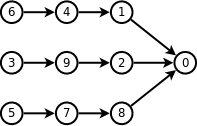# 拓扑排序入门（真的很简单）//b[]为每个点的入度
for(i=1;i<=n;i++){
for(j=1;j<=n;j++){
if(b[j]==0){   //找到一个入度为0的点
ans=j;
vis[cnt++]=j;
b[j]--;
break;
}
}
for(j=1;j<=n;j++)
if(a[ans][j]) b[j]--; //与入度为0的点相连的点的入度减一
}
printf("%d",vis);
for(i=1;i<cnt;i++) printf(" %d",vis[i]);
printf("\n");


    queue<int>q;
vector<int>edge[n];
for(int i=0;i<n;i++)  //n  节点的总数
if(in[i]==0) q.push(i);  //将入度为0的点入队列
vector<int>ans;   //ans 为拓扑序列
while(!q.empty())
{
int p=q.front(); q.pop(); // 选一个入度为0的点，出队列
ans.push_back(p);
for(int i=0;i<edge[p].size();i++)
{
int y=edge[p][i];
in[y]--;
if(in[y]==0)
q.push(y);
}
}
if(ans.size()==n)
{
for(int i=0;i<ans.size();i++)
printf( "%d ",ans[i] );
printf("\n");
}
else printf("No Answer!\n");   //  ans 中的长度与n不相等，就说明无拓扑序列

#include<bits/stdc++.h>
using namespace std;
typedef long long LL;
const int inf=1e9;
const int maxn=1e6+5;
vector<int>edge;
int in;
int main()
{
char s;
set<int>k;
while(cin>>s)
{
k.insert(s-'A');
k.insert(s-'A');
if(s=='>')
{
in[s-'A']++;
edge[s-'A'].push_back(s-'A');
}
else
{
in[s-'A']++;
edge[s-'A'].push_back(s-'A');
}
}
priority_queue<int,vector<int>,greater<int> >q;
for(int i=0;i<30;i++)
{
if(in[i]==0&&k.count(i)!=0)
q.push(i);
}
vector<int>ans;
while(!q.empty())
{
int p=q.top(); q.pop();
ans.push_back(p);
for(int i=0;i<edge[p].size();i++)
{
int y=edge[p][i];
in[y]--;
if(in[y]==0&&k.count(y)!=0)
q.push(y);
}
}
if(ans.size()==k.size())
{
for(int i=0;i<ans.size();i++)
printf("%c",ans[i]+'A');
printf("\n");
}
return 0;
}

HDU-4857 点击打开链接

# 逃生

Time Limit: 2000/1000 MS (Java/Others)    Memory Limit: 32768/32768 K (Java/Others)
Total Submission(s): 6725    Accepted Submission(s): 1965

Problem Description

Input

Output

Sample Input

 

15 103 51 42 51 23 41 42 31 53 51 2

Sample Output

 

1 2 3 4 5#include<iostream>
#include<cstdio>
#include<cstring>
#include<algorithm>
#include<vector>
#include<queue>
using namespace std;
typedef long long ll;
vector<int>edge,ans;
priority_queue<int>q;
int in;
int T,n,m;
void init()
{
for(int i=1;i<=n;i++)
{
edge[i].clear();
in[i]=0;
}
while(!q.empty()) q.pop();
ans.clear();
}
void solve()
{
int i,j;
for(i=1;i<=n;i++)
if(in[i]==0) q.push(i);
while(!q.empty())
{
int p=q.top(); q.pop();
ans.push_back(p);
for( i=0; i<edge[p].size(); i++ )
{
int v=edge[p][i];
in[v]--;
if(in[v]==0) q.push(v);
}
}
for(i=ans.size()-1;i>0;i--)
printf("%d ",ans[i]);
printf("%d\n",ans);
}
int main()
{
int a,b;
scanf("%d",&T);
while(T--)
{
scanf("%d%d",&n,&m);
init();
while(m--)
{
scanf("%d%d",&a,&b);
edge[b].push_back(a);
in[a]++;
}
solve();
}
return 0;
}


10-06
08-09359210-271947
02-041万+
04-211万+
05-071881
07-281202
04-16190
02-243951
05-27199
07-162万+
04-032856
05-1475
03-104752
11-031733
05-181396
03-311万+
11-02204
08-03
04-091380点击重新获取扫码支付余额充值